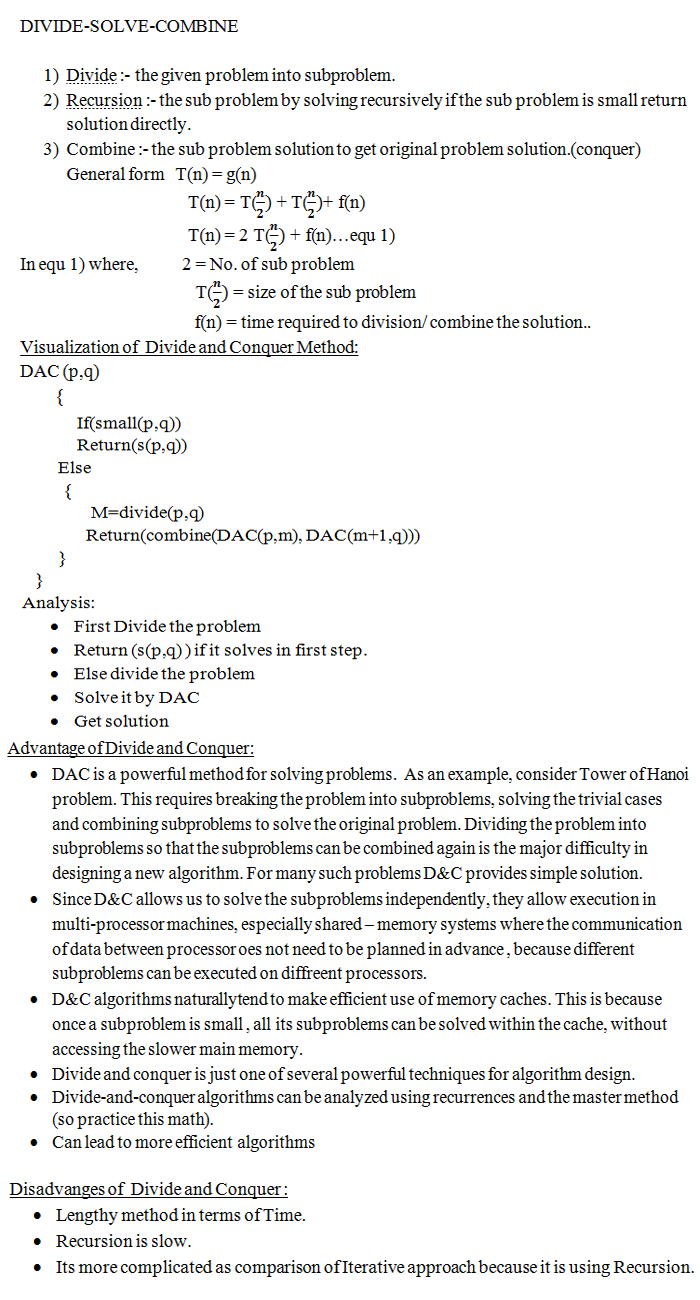## DESIGN AND ANALYSIS OF ALGORITHM

lean basics of Divide and Conquer method, Application based on Divide and Conquer
25 December, 2016

Divide and Conquer Method-  This is the very important method to solve problems in Algorithms. It is basically based on Recursion which we alredy learnt earlier.

What is DIVIDE AND CONQUER Strategy?

D&C algorithms works by recursively breaking down a problem into two or more sub problems of the same type.  The solutions to the sub problems are then combined to give a solution to the original problem.   Like in a game when we need to search, we start from one part and solved step by step .  This is the same strategy.

Given a function to compute on n inputs the divide-and-conquer strategy suggest splitting the inputs into k distinct subsets, 1 < K ≤ n, yielding k sub problems. These Sub problems must be solved, and then a method must be found to combine sub solutions into a solution of the whole. If the Sub problems are still relatively large, then the divide-and-conquer strategy can possibly be reapplied. Often the sub problems resulting from a divide-and-conquer design are the same type as the original problem. For those cases the reapplication of the divide-and-conquer principle is naturally expressed by a recursive algorithm. Now smaller sub problems of the same kind are generated until eventually sub problems that are small enough to be solved without splitting are produced.

A divide-and-conquer algorithm for this problem would proceed as follows:  Let P = (n,a[i],….,a[j]) denote an arbitrary instance of the problem. Here n is the number of elements in the list a[i],….,a[j] and we are interested in finding the maximum and minimum of this list. Let small(P) be true when n ≤ 2. In this case, the maximum and minimum are a[i] if n = 1. If n = 2, the problem can be solved by making one comparison.

Some Real life based examples are as follows:

• Finding a path in a game.
Another example like in a phone book,  How we search for a number is using the DAC method,(DivideAndConquer)
• Finding the exit  within a hotel.
• From above it looks very easy method but in our life its everywhere.

Applications of  Divide and Conquer method –

1)    Finding maximum and minimum in given array .
2)    Power of an Integer
3)    Linear search
4)    Binary search
5)    Heap Sort
6)    Bubble Sort
7)    Selection Sort
8)    Insertion Sort
9)    Strassen’s Matrix multiplication
10)    Quick sort
11)    Merge sortIn the next chapter, we will start with Finding Maximum and Minimum in an array.

U have to learn the concept first, then move to how to solve the problems and designing of Algorithm.

In last find the Time Complexity and other aspects of Problems. Try for the concept and compare it with the Real life problems then You will

find urself in save mode in Algorithm.

Algorithm always help you to learn the basics and through this approach, you will be more reliable on others subjects because it will help

you to understand the all points and subjects.

Till den have fun .. Bye TC..